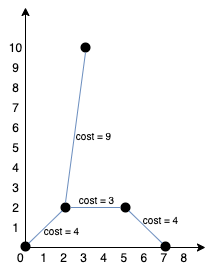# 1584. Min Cost to Connect All Points

Min Cost to Connect All Points - LeetCode

You are given an array points representing integer coordinates of some points on a 2D-plane, where points[i] = [xi, yi]. The cost of connecting two points [xi, yi] and [xj, yj] is the manhattan distance between them: |xi - xj| + |yi - yj|, where |val| denotes the absolute value of val. Return the minimum cost to make all points connected. All points are connected if there is exactly one simple path between any two points.

Example 1:Input: points = [[0,0],[2,2],[3,10],[5,2],[7,0]] Output: 20 Explanation:We can connect the points as shown above to get the minimum cost of 20. Notice that there is a unique path between every pair of points.

Example 2: Input: points = [[3,12],[-2,5],[-4,1]] Output: 18

Constraints:

1 <= points.length <= 1000 -106 <= xi, yi <= 106 All pairs (xi, yi) are distinct.

• code #Prim
``````class Solution:
def minCostConnectPoints(self, points: List[List[int]]) -> int:
def dis(pi, pj):
return abs(pi - pj) + abs(pi - pj)

cost = 0
n = len(points)
visited = [False] * n

cur = 0 # start from point 0
unconnected = n - 1 # point 0 as connected and visited
visited = True

pq = []
heapq.heapify(pq)
while unconnected > 0:
for point in range(n):
if not visited[point]: # from current, to every unvisited point
heapq.heappush(pq, (dis(points[cur], points[point]), point))

while pq:
shortest, j = heapq.heappop(pq)
if not visited[j]: # j is the shortest unvisited point
break

unconnected -= 1
cost += shortest
visited[j] = True
cur = j # update to next round

return cost
``````
• code #Prim shorter, optimized
``````class Solution:
def minCostConnectPoints(self, points: List[List[int]]) -> int:
def dis(pi, pj):
return abs(pi - pj) + abs(pi - pj)

cost = 0
n = len(points)
visited = set()
pq = [[0, 0]] # (distance, current point)

while len(visited) < n:
shortest, cur = heapq.heappop(pq)
if cur in visited: continue

cost += shortest

for point in range(n):
if point not in visited: # from current, to every unvisited point
heapq.heappush(pq, (dis(points[cur], points[point]), point))

return cost
``````
• code #Kruskal #unionfind
``````class Solution:
def minCostConnectPoints(self, points: List[List[int]]) -> int:
n = len(points)
root = [i for i in range(n)]
rank =  * n

def find(x):
if x != root[x]:
root[x] = find(root[x])
return root[x]

def union(x, y):
rtx = find(x)
rty = find(y)
if rtx == rty: return False
rkx = rank[rtx]
rky = rank[rty]
if rkx > rky:
root[rty] = rtx
elif rkx < rky:
root[rtx] = rty
else:
root[rty] = rtx
rank[rtx] += 1
return True

def dis(px, py):
return abs(px - py) + abs(px - py)

dis_pq = []
for i in range(n-1):
for j in range(i+1, n):
dis_pq.append((dis(points[i], points[j]), i, j)) # put all edges

heapq.heapify(dis_pq)
res = 0
while n - 1 > 0:
cur_dis, i, j = heapq.heappop(dis_pq)
if union(i, j): # if can connect, means not already connected, not a cycle
res += cur_dis
n -= 1

return res
``````